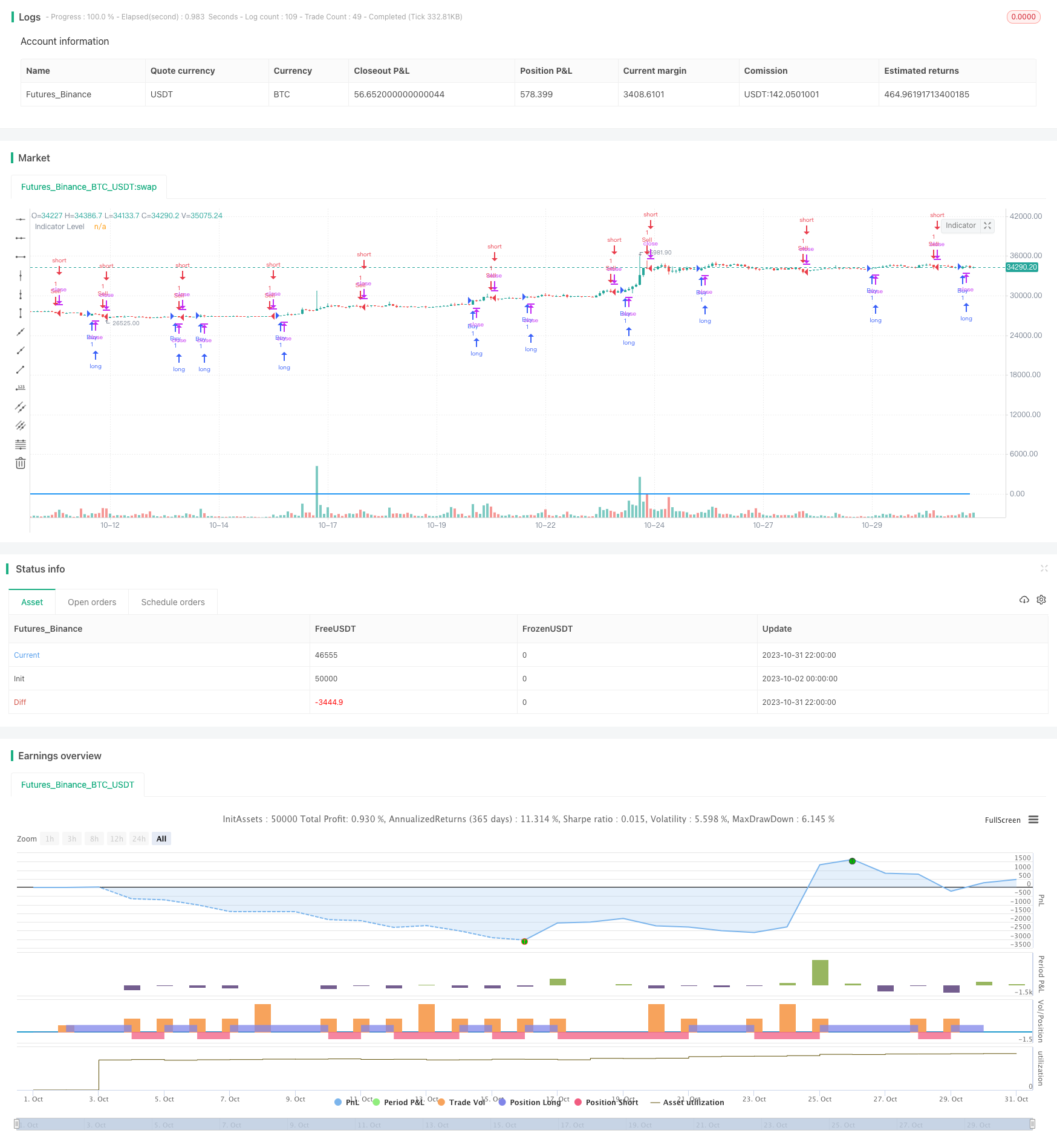# Stochastic RSI and Volume Based Trading Strategy

Author: ChaoZhang, Date: 2023-11-02 14:12:13
Tags:### Overview

This strategy combines the Stochastic RSI indicator and trading volume. It generates buy and sell signals when the Stochastic RSI indicator crosses over, and only trades when the volume is higher than the average volume of the past 7 days. The goal is to identify overbought and oversold conditions using the StochRSI indicator, and then filter false signals using volume, to find trading opportunities during strong trends.

### Strategy Logic

Firstly, the 14-period RSI is calculated, and then the Stochastic indicator is applied on the RSI to generate the StochRSI K and D values. The StochRSI indicator signals overbought and oversold conditions.

Then, the difference between the K and D values are computed. When the difference is above 0, the indicator level is set to 1, and when below 0, it is set to -1. The indicator level determines the long/short state of the StochRSI.

Next, the average volume over the past 7 days is calculated. When the K value crosses above the D value (indicator level changes from negative to positive), and the close is higher than open, and volume is greater than average, it signals a buy. When K crosses below D (indicator level changes from positive to negative), and close is lower than open, and volume is greater than average, it signals a sell.

So in summary, the strategy combines the StochRSI indicator to identify overbought/oversold conditions, and volume to filter out false signals, to trade during strong trends.

1. StochRSI identifies overbought/oversold levels for mean reversion trades. Volume avoids false signals during range-bound markets.

2. Volume condition filters out low volume false breakouts. Trading only during high volume trends improves profitability.

3. Combination of K/D crossovers and volume provides robust signals, avoiding false signals.

4. Simple and easy to understand logic, suitable for algo trading implementation.

### Risk Analysis

1. StochRSI can lag K/D crossovers. Parameters need optimization for sensitivity.

2. Volume amplification may cause huge losses during market crashes. Need stop loss to control risk.

3. Over-reliance on StochRSI may cause issues with false breakouts. Needs more logic.

4. Volume filter may miss some trading opportunities. Can add analysis of ticks, tick power for optimization.

### Optimization Directions

1. Optimize StochRSI parameters to find best K, D values for sensitivity.

2. Add moving average of volume to determine volume trends, avoiding false signals when volume drops.

3. Add other indicators like MACD, RSI for combo signals to improve accuracy.

4. Add stop loss based on ATR for dynamic stop loss management.

5. Analyze parallel and contrary volume to avoid excessive risk from parallel volume.

6. Use adaptive StochRSI parameters based on market regime.

### Conclusion

This strategy primarily utilizes the StochRSI to determine overbought/oversold and the K/D crossovers for signals. It adds volume analysis to filter false signals and trade only during strong trends. The simple integration of indicators creates an easy to implement algo strategy. Further testing and optimization can improve robustness and profitability. However volume amplification risks need to be monitored and stop losses are recommended to control risk.

```/*backtest
start: 2023-10-02 00:00:00
end: 2023-11-01 00:00:00
period: 2h
basePeriod: 15m
exchanges: [{"eid":"Futures_Binance","currency":"BTC_USDT"}]
*/

//@version=5
strategy("StochRSI Volume Strategy", overlay = true)

// StochRSI inputs
smoothK = input.int(3, title="K")
smoothD = input.int(3, title="D")
lengthRSI = input.int(14, "RSI Length")
lengthStoch = input.int(14, "Stochastic Length")

// Calculate StochRSI
rsiValue = ta.rsi(close, lengthRSI)
k = ta.sma(ta.stoch(rsiValue, rsiValue, rsiValue, lengthStoch), smoothK)
d = ta.sma(k, smoothD)

// Calculate difference between lines
lineDifference = k - d

// Calculate indicator level based on line positions
level = lineDifference >= 0 ? 1 : -1

// Calculate mean of last 7 volume bars
meanVolume = ta.sma(volume, 7)

// Determine buy and sell conditions
buyCondition = level > -1 and level <= -1 and close > open and volume > meanVolume
sellCondition = level < 1 and level >= 1 and close < open and volume > meanVolume

// Execute buy and sell signals